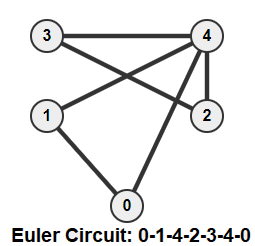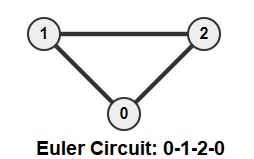# Eulerian Path and Circuit for undirected graphs in C++

In this tute, we will discuss Eulerian Path and Circuit for undirected graphs in C++. Many of us would’ve heard of this somewhere when learning math concepts.

#### What is an undirected graph?

A Graph is a set collection of vertices(points) and edges(lines connecting two points). The graph in which the edge can be traversed in both directions is called an Undirected graph.

## Eulerian Path

A Eulerian Path is a path in the graph that visits every edge exactly once. The path starts from a vertex/node and goes through all the edges and reaches a different node at the end. There is a mathematical proof that is used to find whether Eulerian Path is possible in the graph or not by just knowing the degree of each vertex in the graph.

The necessary conditions are:

1. Firstly, all the vertices with degree >0 should be in the same component (connected).
2. Secondly, only two edges must have an odd degree and all the remaining edges should have even degrees.

Let’s study the case in detail. Firstly, the graph always has an even degree because, in an undirected graph, each edge adds 2 to the overall degree of the graph. So, there should be an even number of odd degree vertices. In this case, let’s consider the graph with only 2 odd degrees vertex.

Let’s start from one of the odd (degree) vertex and go through the remaining edges. When entering a vertex, the degree is reduced by 1, and while exiting, it reduces by 1 again. So, every intermediate vertex must have an even degree. The start and end nodes have to do one of the two operations. Hence, they’ll have an odd degree.

Below is an example diagram of Euler path## Eulerian Circuit

A Eulerian circuit is a Eulerian path in the graph that starts and ends at the same vertex. The circuit starts from a vertex/node and goes through all the edges and reaches the same node at the end. There is also a mathematical proof that is used to find whether a Eulerian Circuit is possible in the graph or not by just knowing the degree of each vertex in the graph.

The necessary conditions are:

1. Firstly, all the vertices with degree >0 should be in the same component (connected).
2. Secondly, all edges must have an even degree.

The proof goes similar to the previous one. So, let’s see the implementation for finding whether the graph has an Euler Path and Circuit.

Examples:Algorithm:

```1. Consider the vertices with degree >0.
2. Check if all those vertices are connected.
3. Look at the degree of all the vertices.
4. If all the vertices have even degree, Euler Circuit is possible.
5. If only 2 vertices have odd degree, Euler Path is possible.```

Code:

```#include<bits/stdc++.h>
using namespace std;

vector<int> g;

{
g[from].push_back(to);
g[to].push_back(from);
}

void dfs(int cur, bool visited[])
{
visited[cur] = true;

}

bool isConnected(int n, bool visited[])
{
int start=-1;

for(int i=0 ; i<n ; ++i)
{
if(!visited[i] && g[i].size() > 0)
{
if(start == -1)             // First component
{
dfs(i, visited);
start = i;
}
else                        // Second component
{
cout<<"The graph is not Eulerian"<<endl;
return false;
}
}
visited[i] = true;
}

return true;                        // 0 or 1 Component
}

void checkGraph(int n)
{
bool visited[n];
fill(visited, visited+n, false);

if(isConnected(n, visited))
{
int oddV = 0;                   // Count odd degree vertices
for(int i=0 ; i<n ; ++i)
if(g[i].size()%2 == 1)
oddV++;

if(oddV == 0)
cout<<"The graph has Euler Circuit"<<endl;
else if(oddV == 2)
cout<<"The graph has Euler Path"<<endl;
else
cout<<"The graph is not Eulerian"<<endl;
}
}

int main()
{
for(int i=0 ; i<5 ; ++i) g[i].clear();  // Clears all the edges

checkGraph(5);

for(int i=0 ; i<5 ; ++i) g[i].clear();  // Clears all the edges

checkGraph(5);

for(int i=0 ; i<5 ; ++i) g[i].clear();  // Clears all the edges

checkGraph(5);

for(int i=0 ; i<3 ; ++i) g[i].clear();  // Clears all the edges

checkGraph(3);

for(int i=0 ; i<3 ; ++i) g[i].clear();  // Clears all the edges

checkGraph(3);

return 0;
}```

Output:

```The graph has Euler Path
The graph has Euler Circuit
The graph is not Eulerian
The graph has Euler Circuit
The graph has Euler Circuit```

Time Complexity:

The time complexity is O(V + E).

Finally, If you have any queries or doubts related to Eulerian Path and Circuit for undirected graphs in C++, simply comment in the comment section provided below.